600700800830100011001200140015001600610×460×540800×500×1900800×470×5001000×580×1900910×505×840605×465×1850825×465×18501000×520×19001000×520×1950825×465×19001000×520×1850260×166×8001200×800×800700×500×1950910×520×19001126×530×20001200×150×7001200×600×1900700×530×1900800×475×19001000×580×8401180×590×8101524×590×810250×190×960570×800×900600×140×700605×465×1800780×570×820800×480×540800×500×1840800×520×1850800×550×800810×525×1950825×465×18001000×450×20001000×550×19001000×600×19001005×525×19001166×581×9001170×585×8001190×595×22001200×550×8501200×560×8501200×600×8501210×510×8751210×530× 19501220×590×8101260×576×20280×140×772400×700×125450×700×140600×450×1900600×480×1850605×460×1900605×465×1900660×483×510750×138×700750×140×700780×590×810810×460×1900810×525×2000870×585×800880×590×810900×490×1850954×576×20980×5501000×470×5001000×480×5401000×480×5601000×500×8001000×500×8801000×520×8501000×550×8301000×570×8001010×533×19501020×465×5401020×483×5101030×605×8201200×600×8701200×600×9681204×462×500260×140×800300×150×800500×398×480568×470×500600×440×550600×470×500600×480×1900700×470×500700×490×1850730×485×786762×590×810775×350×770800×460800×477×1900800×480×480800×480×560800×480×840800×520×1950800×600×850810×465×540900×530×2000900×570×800904×462×500910×465×540990×595×21101000×450×18501000×450×19001000×450×3201000×470×8001000×470×8501000×500×4801000×500×8301000×500×8401000×500×8551000×520×18001000×535×8201000×540×18001000×550×5001000×550×8501000×560×8401000×560×8501000×590×19501000×590×7891000×595×22001000×600×20801000×600×21501000×600×8501000×600×8701010×530×19001010×535×20001010×535×20201040×590×20001065×580×9001066×590×8101100×480×5201100×580×21301190×595×22051190×595×23001200×590×19501200×590×7891200×590×9651200×600×20801200×600×21501200×600×9201200×615×22001200×800×600120×520×8301210×465×5401220×550×7001220×556×8251220×556×8261250×580×8601300×550×8401300×580×21301490×595×22051500×560×8501500×600×21501500×600×21801500×600×22001600×590×7891640×590×20001975×500×515270×144×530280×140×800280×140×900300×140×800300×155×800300×165×800300×200×1000390×495×1150395×416×500400×200×1400440×150×1920440×150×807550×416×500550×440×500550×500×855550×540×888560×370×815570×500×785572×435×500584×476×833600×460600×460×500600×510×530610×460×830615×490×520660×490×765660×700×125680×560×810700×148×800700×150×800700×460700×460×530700×465×1850700×480×840710×460×460715×490×520745×460×500750×455×490750×500×1900760×280×170772×435×500776×438×540790×595×2110790×595×2160790×595×2200795×536×1900800×400×1458800×450×520800×460×500800×470×800800×470×820800×480800×480×500800×480×515800×480×530800×480×850800×490800×520×1912800×530×1900800×550×1200800×590×850800×600×2120810×514×2000810×525×1900815×490×520815×585×1900820×510×750825×467×1900840×595×2200850×590×810870×475×500872×435×500875×500×515896×567×834900×500×880900×510×530900×520×1950900×530×1900900×550×840900×580×2030902×570×20905×480×630914×556×825914×556×826914×590×810930×490×786964×360×800965×580×900972×435×500976×438×540990×560×810990×590×810990×595×2200D170×H800×W900L×W×7001000×390×5201000×4001000×470×3531000×470×8201000×470×8301000×4801000×480×3801000×480×3831000×480×4801000×480×5001000×480×5201000×480×8351000×480×8501000×480×8601000×490×5981000×500×18501000×500×19801000×500×4001000×500×4601000×500×5001000×500×5101000×500×8201000×500×8441000×500×8501000×510×10301000×510×19001000×510×7501000×510×7601000×510×8601000×515×19001000×520×1801

8012035035938040041542044045046046547048049550051051852553054054555056056958059059559960060261061564065066068070072073574075076076576877078078679080080881081581982083083284084585085585786690092094599510001010110012001300143014501476163517501850190019503000

260320350360460520530545550559560570580590594595600605610615620660680700710715720730740744750755760761770780790795798800804810815820825830838844860870880890894895900908910922925930944950954960970975980100010101015105010801107112011301180119812001214123012401330138015001600193025204300

2020201920182017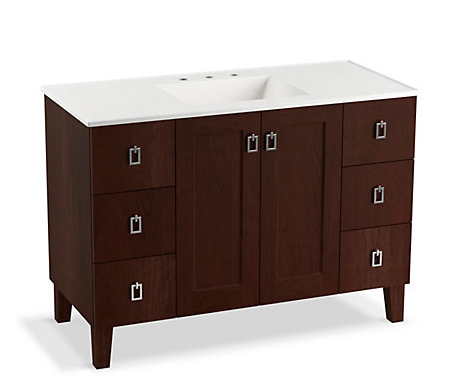13%
2020/10/18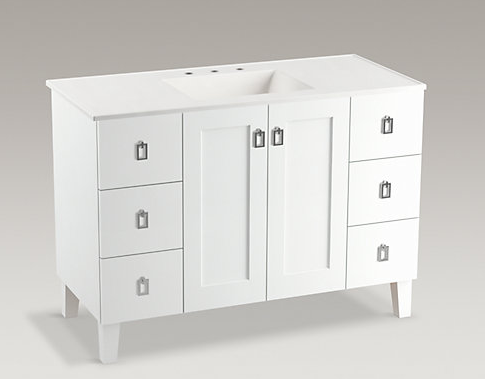13%
2020/10/18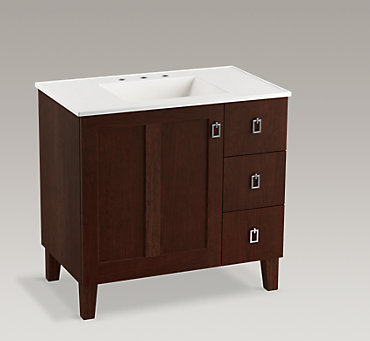13%
2020/10/18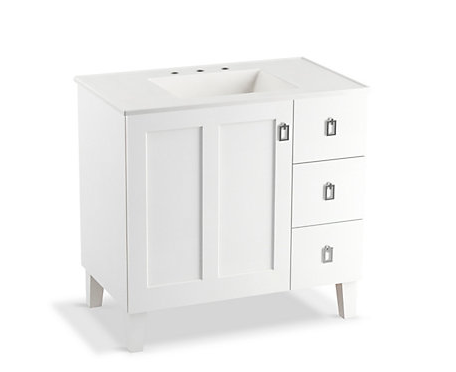13%
2020/10/18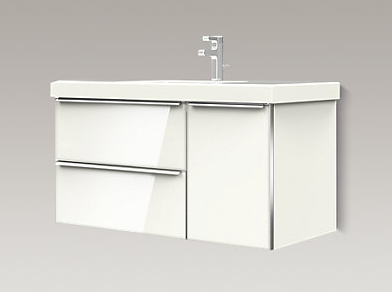13%
2020/10/18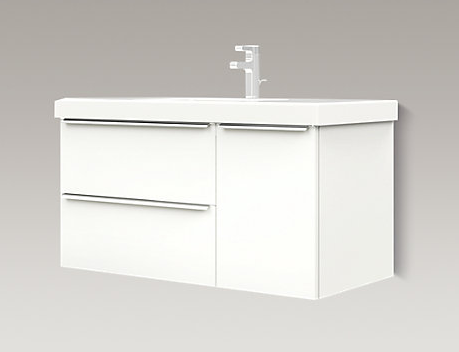13%
2020/10/18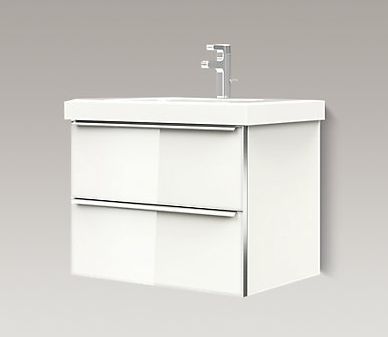13%
2020/10/18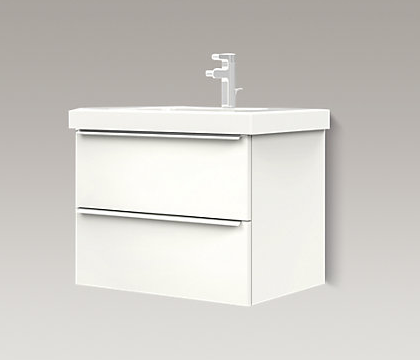13%
2020/10/18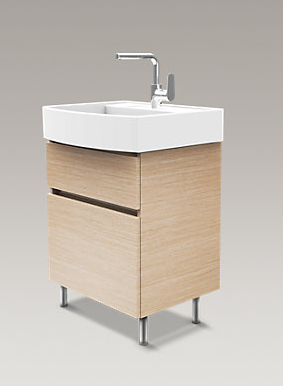13%
2020/10/1813%
2020/10/18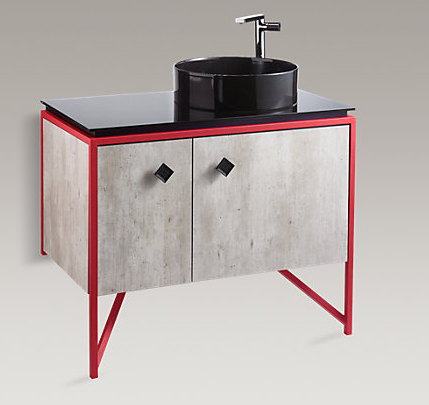13%
2020/10/18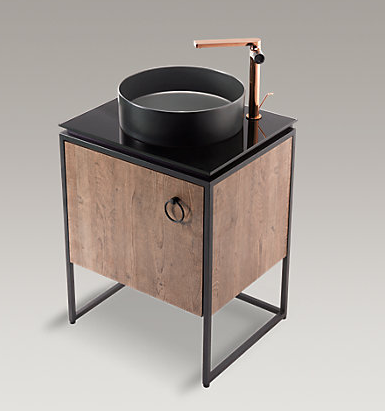13%
2020/10/18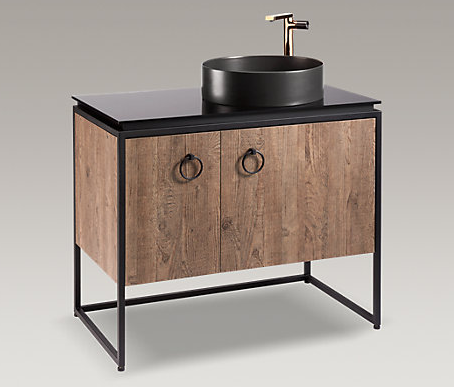13%
2020/10/18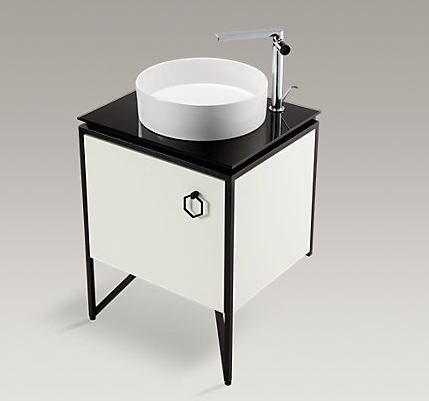13%
2020/10/18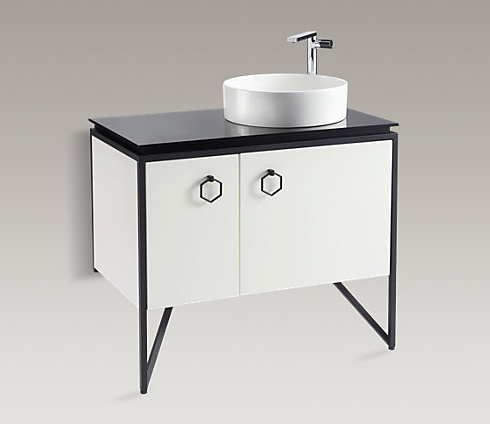13%
2020/10/18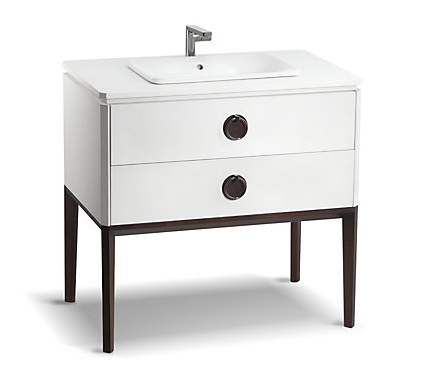13%
2020/10/18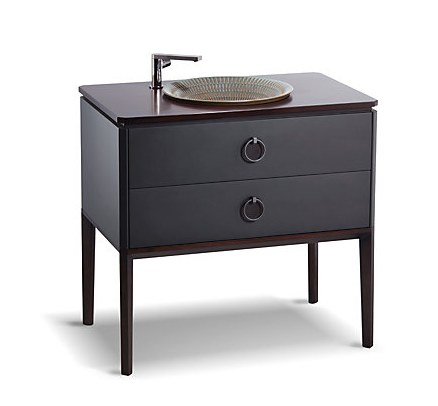13%
2020/10/18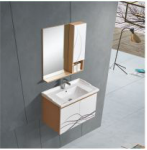13%
2020/10/1513%
2020/10/1513%
2020/10/1513%
2020/10/1513%
2020/10/1513%
2020/10/1513%
2020/10/1513%
2020/10/1513%
2020/10/1513%
2020/10/1513%
2020/10/1513%
2020/10/1513%
2020/10/1513%
2020/10/1513%
2020/10/1513%
2020/10/1513%
2020/10/1513%
2020/10/1513%
2020/10/1513%
2020/10/1513%
2020/10/1513%
2020/10/1513%
2020/10/15

• 1
• 2
• 3
• 4
• 5
• 6
• 100订阅中心广联达大数据